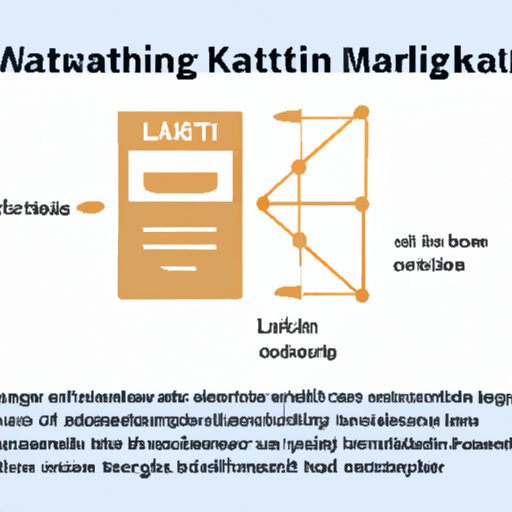# What Math is Needed for Data Science? Exploring the Mathematical Foundations of the Field## Introduction

Data science has become an increasingly popular field in recent years, with many people seeking out ways to use data to understand the world around them. But what math is needed for data science? To be a successful data scientist, it’s important to have a strong understanding of the mathematical foundations of the field. This article will explore the mathematics necessary for data science, from statistics and probability to linear algebra and calculus.

## Exploring the Mathematical Foundations of Data Science

Data science relies heavily on mathematics to analyze and interpret data. The most important mathematical concepts for data science include statistics, probability, linear algebra, and calculus. Each of these topics has its own unique applications in data science.

## Understanding the Role of Statistics in Data Science

Statistics is one of the most important mathematical tools for data science. At its core, statistics is the study of data. It is used to collect, organize, analyze, and interpret data. Statistics can be divided into two main categories: descriptive and inferential. Descriptive statistics are used to summarize and describe data, while inferential statistics involve making predictions and drawing conclusions based on data. Estimation theory is also important in data science, as it is used to make estimates about the population based on a sample.

## Analyzing Probability and Its Applications in Data Science

Probability is another key concept in data science. It is used to measure the likelihood of certain events occurring. Probability is divided into two main categories: discrete and continuous. Discrete probability distributions are used to model events that can be counted, such as the number of heads in a coin toss. Continuous probability distributions are used to model events that can take any value on a range, such as the height of a person. Bayes’ theorem is also important in data science, as it is used to update probabilities based on new information.

## Investigating Linear Algebra and Its Use in Data Science

Linear algebra is another important mathematical tool for data science. It is used to solve systems of linear equations, which are equations involving multiple variables. Linear algebra also involves vector spaces, which are used to represent data points in multiple dimensions. Matrix algebra is also important in data science, as it is used to represent relationships between variables. Multivariate analysis is also important, as it is used to analyze data sets with multiple variables.

## Examining Calculus and Its Relevance to Data Science

Calculus is another key mathematical tool for data science. Differential calculus is used to analyze how functions change over time, while integral calculus is used to calculate the area under curves. Optimization theory is also important in data science, as it is used to find the best solution to a problem given certain constraints.

## Exploring Machine Learning and Its Role in Data Science

Machine learning is a subset of artificial intelligence (AI) that enables computers to learn and improve from experience without being explicitly programmed. In data science, machine learning is used to analyze large amounts of data and uncover patterns and trends. There are three main types of machine learning algorithms: supervised learning, unsupervised learning, and reinforcement learning. Supervised learning algorithms are used to predict outcomes based on labeled data, while unsupervised learning algorithms are used to discover patterns in unlabeled data. Reinforcement learning algorithms are used to teach machines to make decisions in complex environments.

## Conclusion

To be a successful data scientist, it’s important to have a strong understanding of the mathematical foundations of the field. This includes statistics, probability, linear algebra, calculus, and machine learning. Statistics is used to collect, organize, analyze, and interpret data, while probability is used to measure the likelihood of certain events occurring. Linear algebra is used to solve systems of linear equations, while calculus is used to analyze how functions change over time. Finally, machine learning is used to uncover patterns and trends in large amounts of data. By understanding these mathematical concepts, data scientists can better understand and analyze data.#### By Happy Sharer

Hi, I'm Happy Sharer and I love sharing interesting and useful knowledge with others. I have a passion for learning and enjoy explaining complex concepts in a simple way.